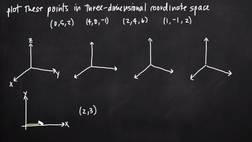PLAY PREVIEW

### Segments in this Video

#### Working with the Third Dimension(04:50)

FREE PREVIEW

In three dimensional coordinate space, points are plotted by moving a distance along the x-axis, y-axis, and z-axis, respectively.

#### Plotting Points in Three-Dimensional Coordinate System (03:43)

To plot points in a three-dimensional coordinate system, locate x on the x-axis, move parallel to the y-axis, move y units, move parallel to the z-axis, then move z units and that will be your point.

#### Illustrate Where the Point is on the Coordinate System(02:12)

To plot points in three-dimensional coordinate space, first graph the points in two dimensions as you would in a standard coordinate plane. Then, use the third dimension to visualize the points in relation to your coordinate axes.

#### Credits(00:00)

Credits

For additional digital leasing and purchase options contact a media consultant at 800-257-5126
(press option 3) or sales@films.com.

# Plotting points in three dimensions

Part of the Series : Integral Calc: Calculus 3
 3-Year Streaming Price: \$49.95

Share

### Description

This video tutorial works through math problems/equations that address topics in Calculus 3, Partial Derivatives. This specific tutorial addresses Plotting points in three dimensions.

Length: 11 minutes

Item#: BVL275743Closed Captioned